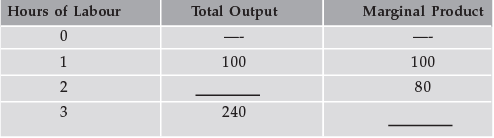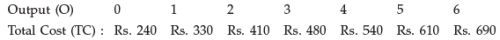Courses

# Test: Theory Of Cost- 4

## 40 Questions MCQ Test Economics for CA CPT | Test: Theory Of Cost- 4

Description
This mock test of Test: Theory Of Cost- 4 for CA Foundation helps you for every CA Foundation entrance exam. This contains 40 Multiple Choice Questions for CA Foundation Test: Theory Of Cost- 4 (mcq) to study with solutions a complete question bank. The solved questions answers in this Test: Theory Of Cost- 4 quiz give you a good mix of easy questions and tough questions. CA Foundation students definitely take this Test: Theory Of Cost- 4 exercise for a better result in the exam. You can find other Test: Theory Of Cost- 4 extra questions, long questions & short questions for CA Foundation on EduRev as well by searching above.
QUESTION: 1

Solution:
QUESTION: 2

Solution:
QUESTION: 3

### Which of the following is not a characteristic of land?

Solution:
QUESTION: 4

Which of the following statements is true?

Solution:
QUESTION: 5

In the production of wheat, all of the following are variable factors that are used by the farmer except :

Solution:
QUESTION: 6

The marginal product of a variable input is best described as:

Solution:
QUESTION: 7

Productivity of land can be raised by:

Solution:
QUESTION: 8

The short run, as economists use the phrase, is characterized by:

Solution:
QUESTION: 9

The marginal, average, and total product curves encountered by the firm producing in the short run exhibit all of the following relationships except:

Solution:
QUESTION: 10

To economists, the main difference between the short run and the long run is that :

Solution:
QUESTION: 11

Which of the following is the best definition of the “production function”?

Solution:
QUESTION: 12

The “law of diminishing returns” applies to :

Solution:
QUESTION: 13

Diminishing returns occur :

Solution:
QUESTION: 14

(Direction 14 - 16) Use the following information to answer questions.Q. What is the total output when 2 hours of labour are employed?

Solution:
QUESTION: 15Q. What is the marginal product of the third hour of labour?

Solution:
QUESTION: 16Q. What is the average product of the first three hours of labour? What is the average product of the first three hours of labour?

Solution:
QUESTION: 17

Which cost increases continuously with the increase in production?

Solution:
QUESTION: 18

Which of the following cost curves is never ‘U’ shaped?

Solution:
QUESTION: 19

Total cost in the short run is classified into fixed costs and variable costs. Which one of the following is a variable cost?

Solution:
QUESTION: 20

In the short run, when the output of a firm increases, its average fixed cost :

Solution:
QUESTION: 21

Which one of the following is also known as planning curve?

Solution:
QUESTION: 22

The cost of one thing in terms of the alternative given up is known as:

Solution:
QUESTION: 23

With which of the following is the concept of marginal cost closely related?

Solution:
QUESTION: 24

Which of the following statements is correct?

Solution:
QUESTION: 25

Which of the following is an example of an “explicit cost”?

Solution:
QUESTION: 26

Which of the following is an example of an “implicit cost”?

Solution:
QUESTION: 27

(Direction 27 - 29) Use the following data to answer questions.Q. The average fixed cost of 2 units of output is  :

Solution:
QUESTION: 28Q. The marginal cost of the sixth unit of output is :

Solution:
QUESTION: 29Q. Diminishing marginal returns start to occur between units :

Solution:
QUESTION: 30

Marginal cost is defined as:

Solution:
QUESTION: 31

Which of the following is true of the relationship between the marginal cost function and the average cost functions?

Solution:
QUESTION: 32

Which of the following statements is true of the relationship among the average cost functions?

Solution:
QUESTION: 33

Which of the following is not a determinant of the firm’s cost functions?

Solution:
QUESTION: 34

Which of the following statements is correct concerning the relationships among the firm’s functions?

Solution:
QUESTION: 35

Suppose output increases in the short run. Total cost will :

Solution:
QUESTION: 36

Which of the following statements concerning the long-run average cost curve is false?

Solution:
QUESTION: 37

The negatively-sloped (i.e. falling) part of the long-run average total cost curve is due to which of the following?

Solution:
QUESTION: 38

The positively sloped (i.e. rising) part of the long run average total cost curve is due to which of the following?

Solution:
QUESTION: 39

A firm’s average total cost is Rs. 300 at 5 units of output and Rs. 320 at 6 units of output.
The marginal cost of producing the 6th unit is :

Solution:

Total cost for producing 5 units = 300 x 5 = 1500

Total cost for producing 6 units = 320 x 6 = 1920

Therefore, Marginal cost for producting the 6th unit =
1920-1500 = 420

QUESTION: 40

A firm producing 7 units of output has an average total cost of Rs. 150 and has to pay Rs. 350 to its fixed factors of production whether it produces or not. How much of the average total cost is made up of variable costs?

Solution: